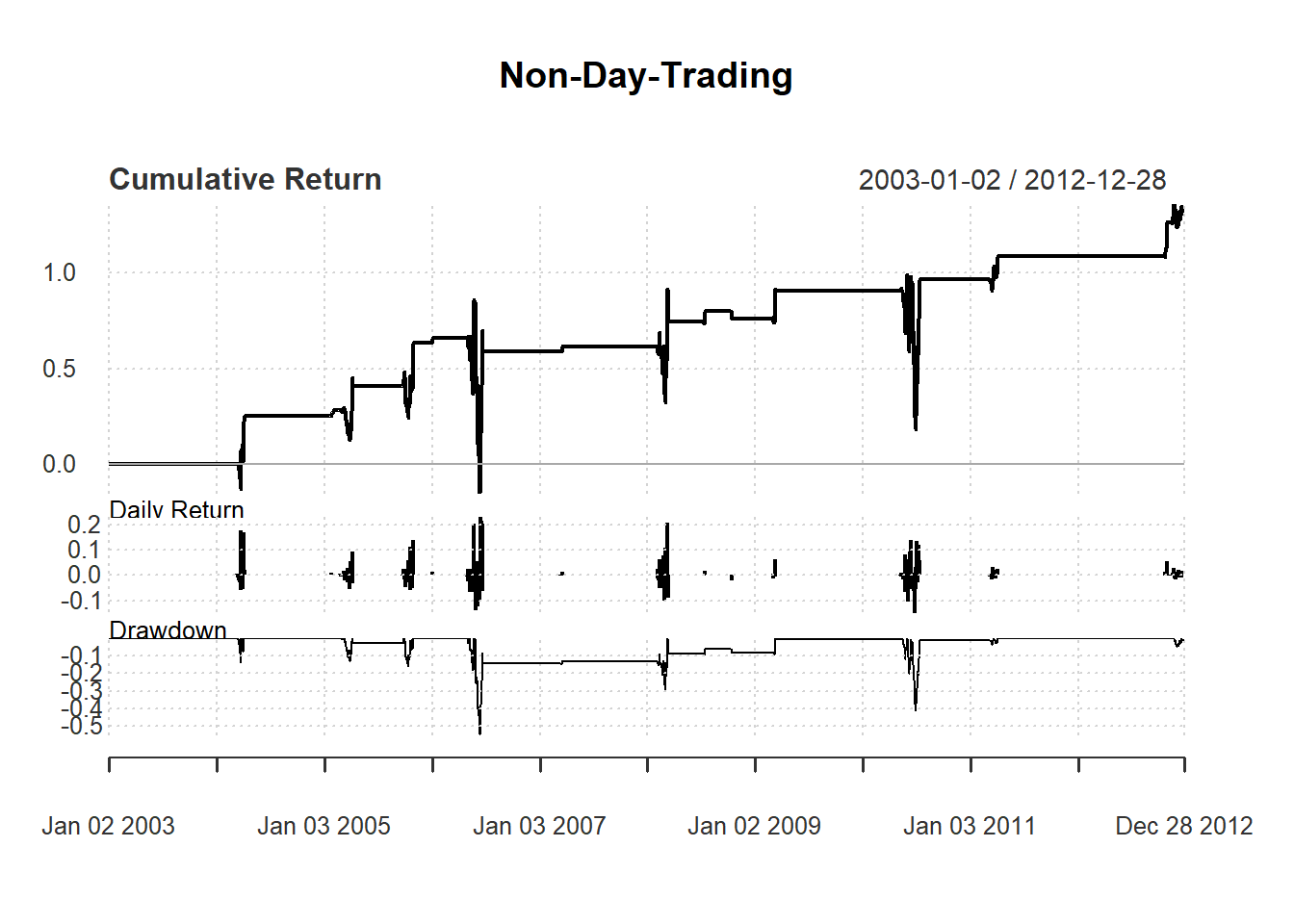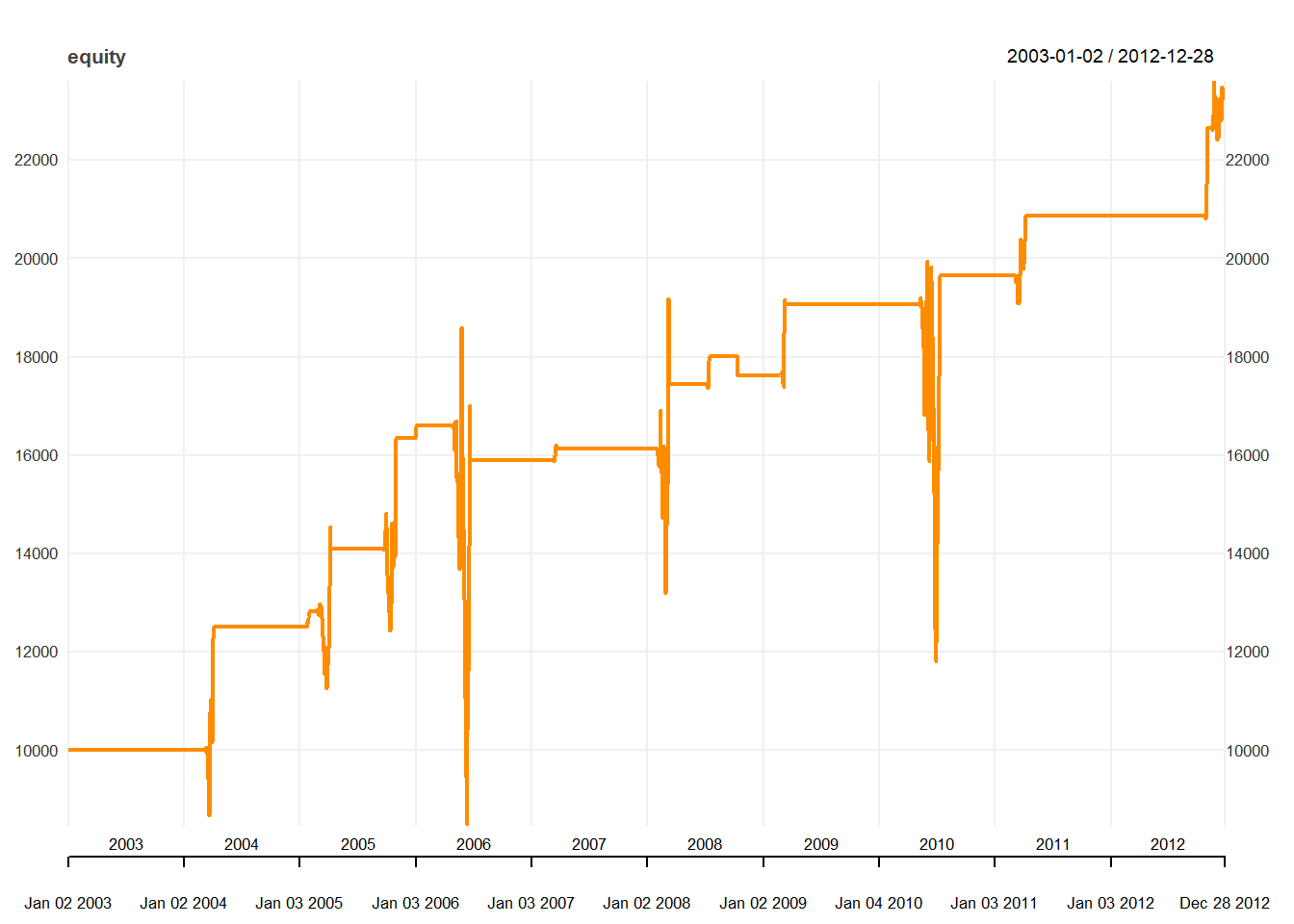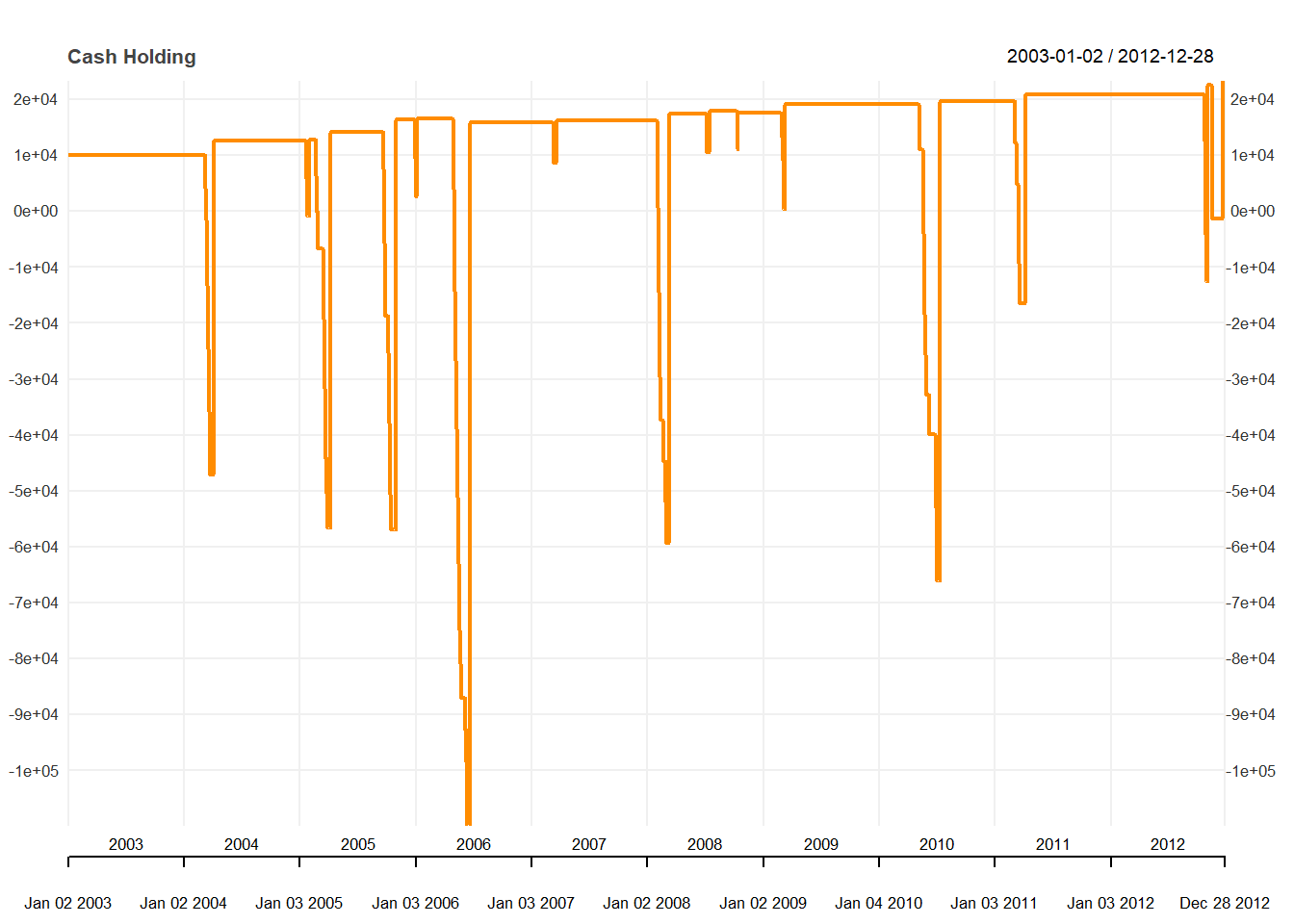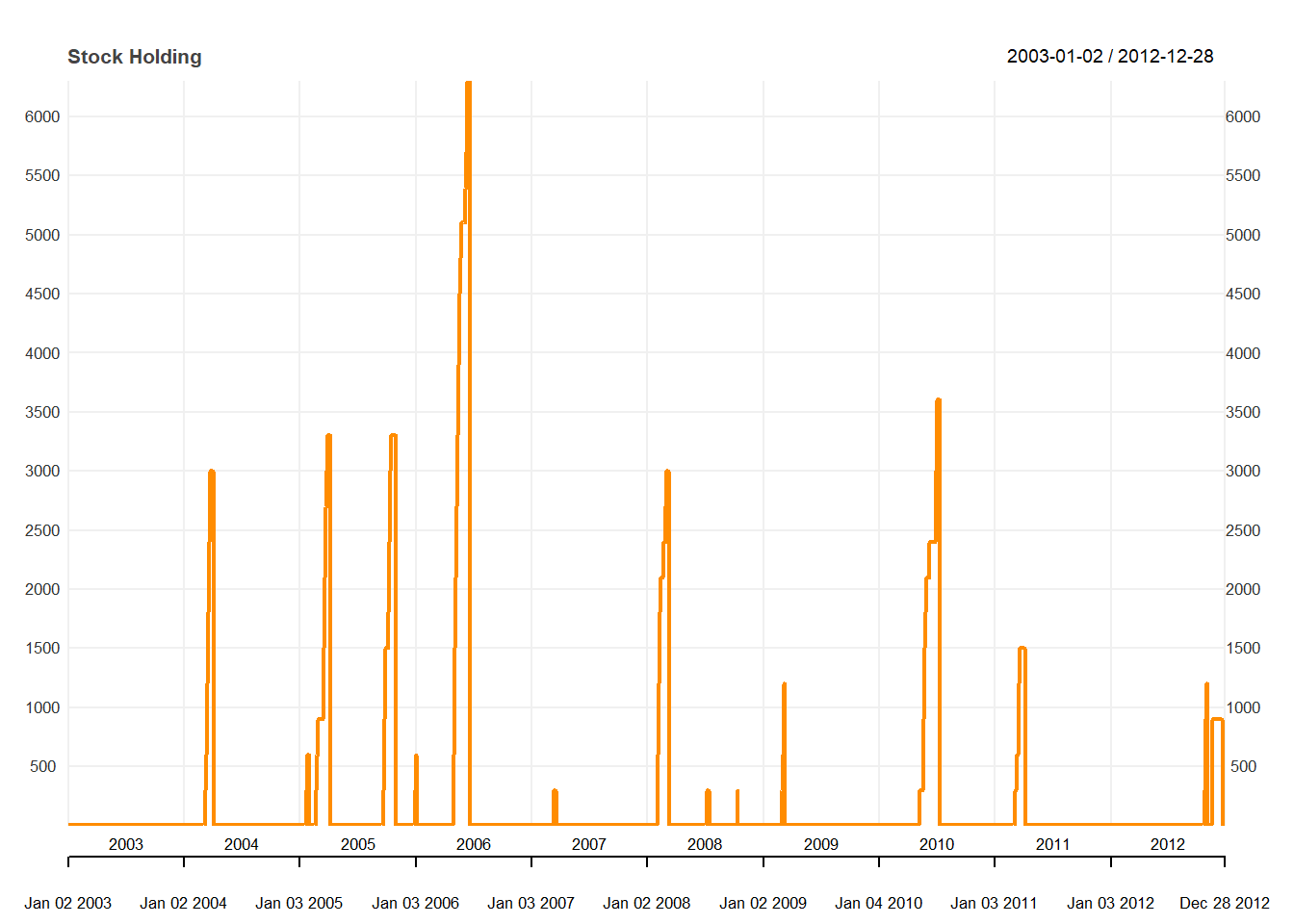• Buy signal arises if 14-day RSI < 30

• Sell signal arises if 14-day RSI > 50

• Sell all when sell signal appears

Initial wealth: 10,000

Note that we need to keep track of both cash and stock holdings.

qty <-300
day <-14

signal[1:(day+1)] <- 0

price <- Cl(MSFT)

stock <- c()  #stock holding
stock[1:(day+1)] <-0

cash <-c()
cash[1:(day+1)] <- 10000  

Trading signal is based on simple RSI:

rsi <- RSI(price, day)  #rsi is the lag of RSI
for (i in (day+1): length(price)){
if (rsi[i] < 30){  #buy one more unit if rsi < 30
signal[i] <- 1
} else if (rsi[i] < 50){ #no change if rsi < 50
signal[i] <- 0
} else {         #sell  if rsi > 50
signal[i] <- -1
}
}
signal<-reclass(signal,price)

Assume buying at closing price. We keep track of how cash and stock changes:

trade <- Lag(signal)    #rsi is the lag of RSI
for (i in (day+1): length(price)){
cash[i] <- cash[i-1] -
} else{
stock[i] <- 0
cash[i] <- cash[i-1] +
stock[i-1]*price[i]
}
}
stock<-reclass(stock,price)
cash<-reclass(cash,price)

To evaluate performance, we calculate equity using cash and stock holdings.

equity <-c()
equity[1:(day+1)] <- 10000

return<-c()
return[1:(day+1)] <- 0

for (i in (day+1): length(price)){
equity[i] <- stock[i] * price[i] + cash[i]
return[i] <- equity[i]/equity[i-1]-1
}
equity<-reclass(equity,price)
return<-reclass(return,price)

Performance Charts

charts.PerformanceSummary(return,
main="Non-Day-Trading")We can plot the equity line showing how the performance of the strategy:

chart_Series(equity, main="equity line")We can check the cash account over time:

chart_Series(cash, name="Cash Holding")Finall, We can stock holdings:

chart_Series(stock, name="Stock Holding")